# NCERT Solutions for Class 10 Maths Chapter 7 Coordinate Geometry Ex 7.2

NCERT Solutions for Class 10 Maths Chapter 7 Coordinate Geometry Ex 7.2 are part of NCERT Solutions for Class 10 Maths. Here are we have given Chapter 7 Coordinate Geometry Class 10 NCERT Solutions Ex 7.2.

 Board CBSE Textbook NCERT Class Class 10 Subject Maths Chapter Chapter 7 Chapter Name Coordinate Geometry Exercise Ex 7.2 Number of Questions Solved 10 Category NCERT Solutions

## NCERT Solutions for Class 10 Maths Chapter 7 Coordinate Geometry Ex 7.2

Question 1. Find the coordinates of the point which divides the join of (- 1, 7) and (4, – 3) in the ratio 2:3.

Solution:
Let P(x, y) be the required point. Using the section formula which says
Coordinates of points P, dividing the line segment joining A(x1, y1) & B(x2,y2)
internally in the ratio m:nConcept Insight: The key idea here is to identify m and n with point A & n with point BQuestion 2. Find the coordinates of the points of trisection of the line segment joining (4, -1) and (-2, -3).

Solution:
Trisection means division into three equal parts. So we need to find two points. such that they divide the line segment in three equal parts.
Let P (x1,y1) and Q (x2,y2) are the points of trisection of the line segment joining the given points i.e. AP = PQ = QB
Therefore point P divides AB internally in ratio 1:2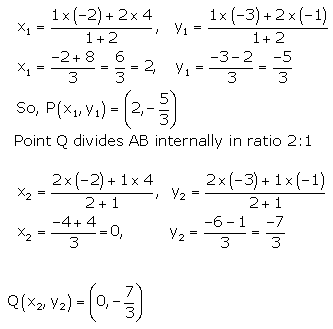Question 3. To conduct Sports Day activities, in your rectangular shaped school ground ABCD, lines have been drawn with chalk powder at a distance of 1 m each. 100 flower pots have been placed at a distance of 1 m from each other along AD, as shown in the following figure. Niharika runs 1/4th the distance AD on the 2nd line and posts a green flag. Preet runs 1/5th the distance AD on the eighth line and posts a red flag. What is the distance between both the flags? If Rashmi has to post a blue flag exactly halfway between the line segment joining the two flags, where should she post her flag?Solution: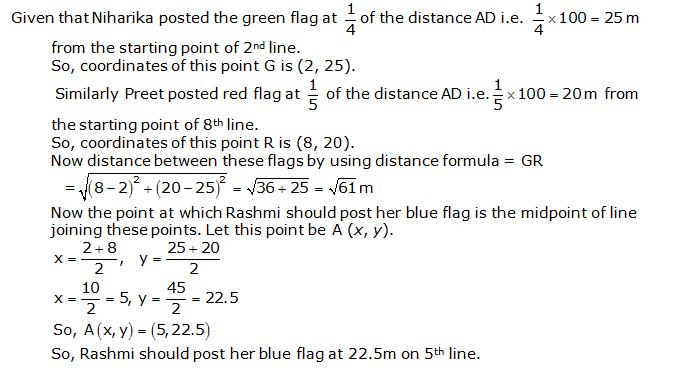Question 4. Find the ratio in which the line segment joining the points (-3, 10) and (6, – 8) is divided by (-1, 6).

Solution:

Let the ratio in which line segment joining (—3, 10) and (6, —8) is divided by
point (—1, 6) is k: 1,Concept Insight: Assume the ratio as k: 1 and not as m :n otherwise we will get one equation in two unknowns.

Question 5. Find the ratio in which the line segment joining A (1, – 5) and B (- 4, 5) is divided by the x-axis. Also find the coordinates of the point of division.

Solution:
If the ratio in which P divides AB is k:1 , then the co-ordinates of the point P will be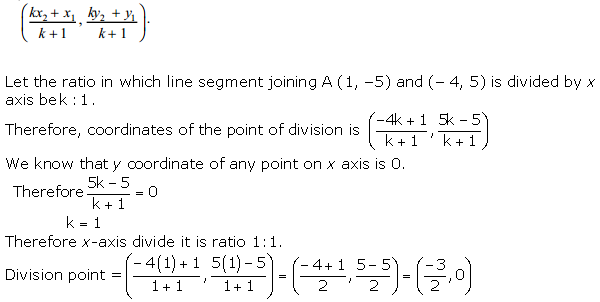Concept Insight: Assume the ratio as k: 1 and not as m :n otherwise we will get one equation in two unknowns.Use the fact that y coordinate is zero.

Question 6. If (1, 2), (4, y), (x, 6) and (3, 5) are the vertices of a parallelogram taken in order, find x and y.

Solution: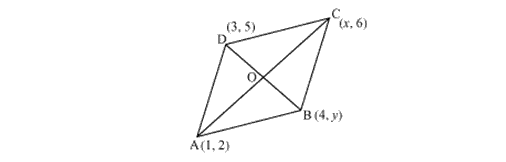Let (1, 2), (4, y), (x, 6) and (3, 5) are the coordinates of A, B, C, D vertices of a parallelogram ABCD.
Diagonals of a parallelogram bisects each other so, O is midpoint of AC and BD
If O is midpoint of AC, then coordinate and O areConcept Insight: To prove that a quadrilateral with given vertices is a parallelogram using prove that the diagonals bisect each other.

Question 7. Find the coordinates of a point A, where AB is the diameter of circle whose centre is (2, – 3) and B is (1, 4).

Solution: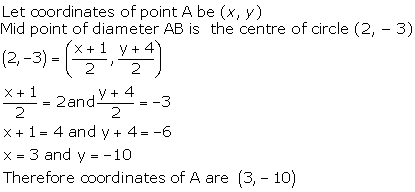Question 8. If A and B are (–2, –2) and (2, –4), respectively, find the coordinates of P such that AP = 3/7 AB and P lies on the line segment AB.

Solution:
Coordinates of the point P(x, y) which divides the line segment joining the points A(x1, y1) and B(x2,y2) intenally in the ratio m1 : m2, are
$$\frac { { m }_{ 1 }{ x }_{ 2 }+{ m }_{ 2 }{ x }_{ 1 } }{ { m }_{ 1 }+{ m }_{ 2 } } ,\frac { { m }_{ 1 }{ y }_{ 2 }+{ m }_{ 2 }{ y }_{ 1 } }{ { m }_{ 1 }+{ m }_{ 2 } }$$Question 9. Find the coordinates of the points which divide the line segment joining A (- 2, 2) and B (2, 8) into four equal parts.

Solution: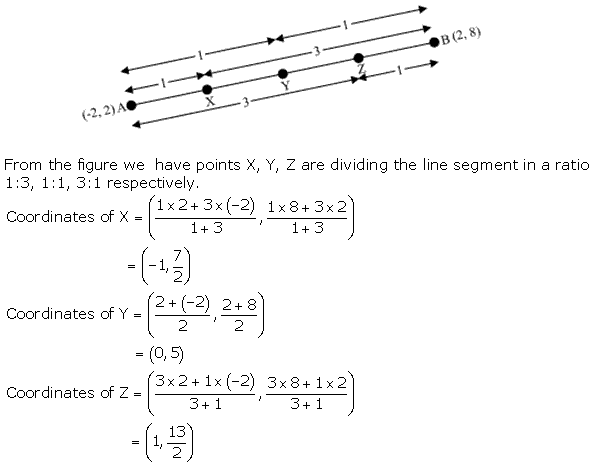Question 10. Find the area of a rhombus if its vertices are (3, 0), (4, 5), (— 1, 4) and (— 2, —1) taken in order.

Solution: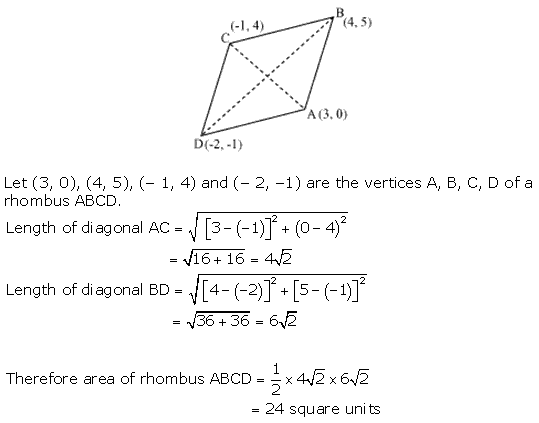Concept Insight: Use the result Area of a rhombus = 1/2 (product of its diagonals) and diagonals are formed by joining opposite vertices.

We hope the NCERT Solutions for Class 10 Maths Chapter 7 Coordinate Geometry Ex 7.2 help you. If you have any query regarding NCERT Solutions for Class 10 Maths Chapter 7 Coordinate Geometry Ex 7.2, drop a comment below and we will get back to you at the earliest.